# Bogolyubov theorem

(diff) ← Older revision | Latest revision (diff) | Newer revision → (diff)

Bogolyubov's edge-of-the-wedge theorem is a generalization of the principle of analytic continuation, in particular to the case of several complex variables. It was obtained in 1956 by N.N. Bogolyubov in the justification of the dispersion relations in quantum field theory (, Appendix A). The modern formulation is as follows. Let a function,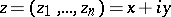, be holomorphic in an open set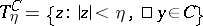, whereis an open cone in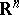with apex at zero such that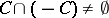, let the open set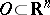be contained in the balland suppose that for any test function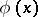from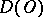the limit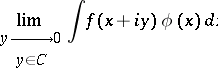exists, independent from the way in which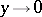,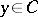; thencan be analytically continued into the domain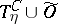:where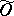is a complex neighbourhood of the set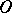,is a constant which depends only on the cone, and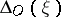is the distance from the pointto the boundary of. Bogolyubov's edge-of-the-wedge theorem also remains valid if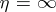. In such a case, and under certain assumptions regarding the growth of the function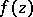, one obtains the original formulation of Bogolyubov (cf. ; the light cone inplays the role of the cone). There exist various proofs and generalizations of this theorem , . In particular, one can mention the generalizations to hyperfunctions  and to holomorphic cocycles .

Bogolyubov's edge-of-the-wedge theorem is extensively employed in axiomatic quantum field theory, in the theory of partial differential equations and in the theory of boundary values of holomorphic functions (especially of functions of several complex variables). A useful completion of the theorem is the-convex hull theorem . Let, under the conditions of Bogolyubov's theorem,,, where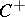is a convex sharp cone; then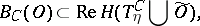where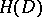is the envelope of holomorphy (cf. Holomorphic envelope) of,is the real section of the domain, and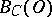is the-convex hull of the set, i.e. the smallest open set containingand having the following property: If the points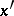and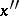ofcan be connected by a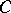-similar curve that is totally contained in, then all-similar curves homotopic to it are located in.

## Contents

How to Cite This Entry:
Bogolyubov theorem. Encyclopedia of Mathematics. URL: http://encyclopediaofmath.org/index.php?title=Bogolyubov_theorem&oldid=16527
This article was adapted from an original article by V.S. Vladimirov (originator), which appeared in Encyclopedia of Mathematics - ISBN 1402006098. See original article